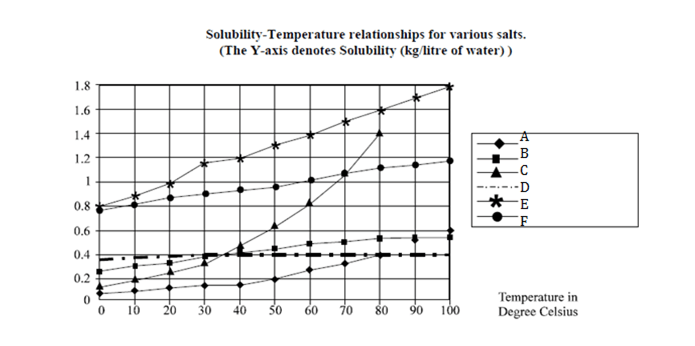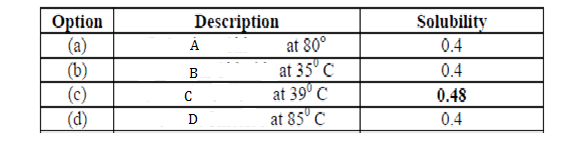Directions for 1 to 4 : are based on the graph given below:Question 1.  Which of the following salts has greatest solubility?
(a) A at 800C.
(b) B at 350 C.
(c) C at 390 C.
(d) D at 850 C.

Question 2.  Approximately, how many kg of C can be dissolved in 10 liters of water at 300C?
(a) 0.04
(b) 0.4
(c) 4
(d) 0.35

Question 3. By what % is the solubility of A in water increased as the water is heated from 300C to 800 C?
(a) 100
(b) 200
(c) 250
(d) 167

Question 4. If 1 mole of B weighs 0.07456 kg, approximately. How many moles of B can be dissolved in 100 liters of water at 360C?
(a) 700
(b) 650
(c) 480
(d) 540

Question 5. Which of the salts has greater change in solubility in kg / liter of water between 150C and 250C?
(a) D
(b) B
(c) E
(d) F

After observing the given line graph, we can make the following deductions;Hence (c) is the correct answer.

The solubility of C in one litter at  300C =  0.38 kg Therefore solubility of C in 10 lt. would be = 3.8 kg., Approx = 4 kg. Therefore the right answer is option (c)

Solubility of A at 30c is .15 while at 80c it is .4 The increase in % of solubility of A = (0.4 – 0.15) 100/0.15 = 167%.